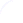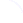Search
 Submission Procedure share: |available in:   PDF (282 kB) PS (87 kB)

get:
 Similar Docs BibTeX Write a comment
get:

DOI:   10.3217/jucs-004-01-0048

### Algebraic Solutions to a Class of Interval Equations

Evgenija D. Popova (Bulgarian Academy of Sciences, Bulgaria)

Abstract: The arithmetic on the extended set of proper and improper intervals is an algebraic completion of the conventional interval arithmetic and thus facilitates the explicit solution of certain interval algebraic problems. Due to the existence of inverse elements with respect to addition and multiplication operations certain interval algebraic equations can be solved by elementary algebraic transformations. The conditionally distributive relations between extended intervals allow that complicated interval algebraic equations, multi-incident on the unknown variable, be reduced to simpler ones. In this paper we give the general type of "pseudo-linear" interval equations in the extended interval arithmetic. The algebraic solutions to a pseudo-linear interval equation in one variable are studied. All numeric and parametric algebraic solutions, as well as the conditions for nonexistence of the algebraic solution to some basic types pseudo-linear interval equations in one variable are found. Some examples leading to algebraic solution of the equations under consideration and the extra func- tionalities for performing true symbolic-algebraic manipulations on interval formulae in a Mathematica package are discussed.

Keywords: Extended interval arithmetic, algebraic solutions, algebraic transformations., interval equation

Categories: G.1.5, I.1.1